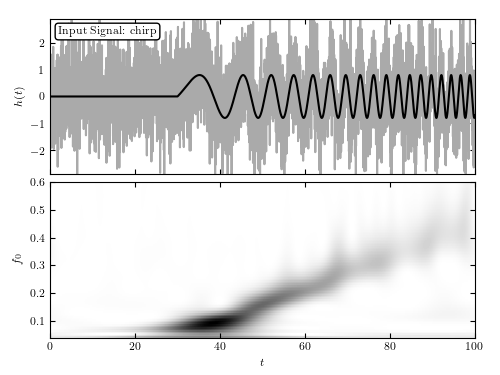# Chirp wavelet PSD¶

Figure 10.28

A wavelet PSD of the ten-parameter chirp signal similar to that analyzed in figure 10.27. Here, the signal with an amplitude of A = 0.8 is sampled in 4096 evenly spaced bins, and with Gaussian noise with sigma = 1. The two-dimensional wavelet PSD easily recovers the increase of characteristic chirp frequency with time.```
```
```# Author: Jake VanderPlas
#   The figure produced by this code is published in the textbook
#   "Statistics, Data Mining, and Machine Learning in Astronomy" (2013)
#   To report a bug or issue, use the following forum:
import numpy as np
from matplotlib import pyplot as plt

from astroML.fourier import FT_continuous, IFT_continuous, wavelet_PSD

#----------------------------------------------------------------------
# This function adjusts matplotlib settings for a uniform feel in the textbook.
# Note that with usetex=True, fonts are rendered with LaTeX.  This may
# result in an error if LaTeX is not installed on your system.  In that case,
# you can set usetex to False.
if "setup_text_plots" not in globals():
from astroML.plotting import setup_text_plots
setup_text_plots(fontsize=8, usetex=True)

#------------------------------------------------------------
# Define the chirp signal
def chirp(t, T, A, phi, omega, beta):
signal = A * np.sin(phi + omega * (t - T) + beta * (t - T) ** 2)
signal[t < T] = 0
return signal

def background(t, b0, b1, Omega1, Omega2):
return b0 + b1 * np.sin(Omega1 * t) * np.sin(Omega2 * t)

np.random.seed(42)
N = 4096
t = np.linspace(-50, 50, N)
h_true = chirp(t, -20, 0.8, 0, 0.2, 0.02)
h = h_true + np.random.normal(0, 1, N)

#------------------------------------------------------------
# Compute the wavelet PSD
f0 = np.linspace(0.04, 0.6, 100)
wPSD = wavelet_PSD(t, h, f0, Q=1.0)

#------------------------------------------------------------
# Plot the  results
fig = plt.figure(figsize=(5, 3.75))

# Top: plot the data
ax.plot(t + 50, h, '-', c='#AAAAAA')
ax.plot(t + 50, h_true, '-k')

ax.text(0.02, 0.95, "Input Signal: chirp",
ha='left', va='top', transform=ax.transAxes,
bbox=dict(boxstyle='round', fc='w', ec='k'))

ax.set_xlim(0, 100)
ax.set_ylim(-2.9, 2.9)
ax.xaxis.set_major_formatter(plt.NullFormatter())
ax.set_ylabel('\$h(t)\$')

# Bottom: plot the 2D PSD
ax.imshow(wPSD, origin='lower', aspect='auto',
extent=[t + 50, t[-1] + 50, f0, f0[-1]],
cmap=plt.cm.binary)

ax.text(0.02, 0.95, ("Wavelet PSD"), color='w',
ha='left', va='top', transform=ax.transAxes)

ax.set_xlim(0, 100)
ax.set_ylim(0.04, 0.6001)
ax.set_xlabel('\$t\$')
ax.set_ylabel('\$f_0\$')

plt.show()
```